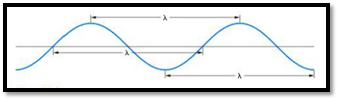# The frequency of the strong red line in the spectrum of potassium is 3.91 × 10 14 /s. What is the wavelength of this light in nanometers?### General Chemistry - Standalone boo...

11th Edition
Steven D. Gammon + 7 others
Publisher: Cengage Learning
ISBN: 9781305580343

#### Solutions

Chapter
Section### General Chemistry - Standalone boo...

11th Edition
Steven D. Gammon + 7 others
Publisher: Cengage Learning
ISBN: 9781305580343
Chapter 7.1, Problem 7.1E
Textbook Problem
121 views

## The frequency of the strong red line in the spectrum of potassium is 3.91 × 1014/s. What is the wavelength of this light in nanometers?

Interpretation Introduction

Interpretation:

The wavelength of red light from the given frequency has to be calculated.

Concept introduction:

Relation between frequency and wavelength is,

C=λν

C is the speed of light.

ν is the frequency.

λ is wavelength.

The distance between any two similar points of a wave is called wavelengthFigure 1

Frequency is defined as number of wavelengths of a wave that can pass through a point in one second.

To calculate: The wavelength of red light from the given frequency.

### Explanation of Solution

Given,

Frequency of red light ν is 3.91×1014/s

C=λνλ=Cν=3

### Still sussing out bartleby?

Check out a sample textbook solution.

See a sample solution

#### The Solution to Your Study Problems

Bartleby provides explanations to thousands of textbook problems written by our experts, many with advanced degrees!

Get Started

Find more solutions based on key concepts
Calorie deficit is no longer the primary strategy for weight management.

Nutrition: Concepts and Controversies - Standalone book (MindTap Course List)

Where are protons, electrons, and neutrons found in an atom?

Biology: The Dynamic Science (MindTap Course List)

A pendulum consists of a small object called a bob hanging from a light cord of fixed length, with the top end ...

Physics for Scientists and Engineers, Technology Update (No access codes included)

Draw the skeleton diagram of 1-bromo-3-methylcyclohexane.

Introductory Chemistry: An Active Learning Approach

What is meant by thermal equilibrium? Is Earths heat budget in balance?

Oceanography: An Invitation To Marine Science, Loose-leaf Versin